# Weight of air

What is the weight of air in the living room measuring width 8 m length 5 m and height 3.1 m? Air density is ρ = 1.2959 kg/m3.

m =  160.7 kg

### Step-by-step explanation: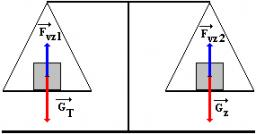Did you find an error or inaccuracy? Feel free to write us. Thank you!Tips to related online calculators
Do you want to convert length units?
Tip: Our volume units converter will help you with the conversion of volume units.
Tip: Our Density units converter will help you with the conversion of density units.

## Related math problems and questions:

• Air massWhat is the weight of the air in a classroom with dimensions 10 m × 10 m × 2.7 m ? The air density is 1.293 kg/m3.
• Cuboid 5Calculate the mass of the cuboid with dimensions of 12 cm; 0.8 dm and 100 mm made from spruce wood (density = 550 kg/m3).
• The glass1 m3 of glass weighs 2600 kg. Calculate the weight of the glass glazing panel with dimensions of 2.5 m and 3.8 m if the thickness of the glass is 0.8 cm
• Wood cuboidWhat is the weight of the wood cuboid 15 cm, 20 cm, 3 m if 1 m3 wood weighs 800 kg?
• An aquariumAn aquarium tank that measures 2.4 m high, 6 m long, and 1.5 m wide and is completely filled with water. The density of water is 1,000 kg/m3. What is the mass of the water in the tank?
• Copper wireWhat is the weight in kg of copper wire 200 m long with a diameter of 0.6 cm if the density of copper is 8.8 kg?
• Sand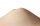How much m ^ 3 of sand can be loaded on a car with a load capacity of 5 t? The sand density is 1600 kg/m3 .
• SandThe maximum weight of the car is 5000 kg. 10 m3 of sand must be transferred. How many times does a car have to go? (density of sand is 1500 kg/m3)
• A shopkeeper 3A shopkeeper sells 8 1/3 kg, 10 1/4 kg and 11 1/5 kg of apples on 3 consecutive days. What is the total weight of apples sold?
• Wood lumberWooden lumber is 4 m long and has a cross section square with side 15 cm. Calculate: a) the volume of lumber b) the weight of the lumber if 1 m3 weighs 790 kg
• Glass doorWhat is the weight of glass door panel 5 mm thick height 2.1 meters and a width of 65 cm and 1 cubic dm of glass weighs 2.5 kg?
• Iron pole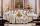What is the mass of a pole with the shape of a regular quadrilateral prism with a length of 1 m and a cross-sectional side length of a = 4.5 cm make from iron with density ρ = 7800 kg/m³?
• Brick wallWhat is the weight of a solid brick wall that is 30 cm wide, 4 m long and 2 m high? The density of the brick is 1500 kg per cubic meter.
• Iron fence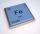One field of iron fence consists of 20 iron rods with square cross-section of 1.5 cm side and a long 1 meter. How much weight a field if the density of iron is 7800 kg/m3.
• Copper plateCalculate the thickness of the copper plate with a density 8.7 g/cm³ measuring 1.5 meters and 80 cm and its weight is 3.65 kg
• Packets weight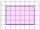The weight of three packets are 3 2/5 kg + 2 1/3 kg + 5 1/2 kg. Find total weight of all the three packets.
• Copper plate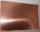Copper plate length 3.2 m and width 50 cm weighs 55.42 kg. What is the plate thick, if 1 m3 of copper weighs about 8700 kg?